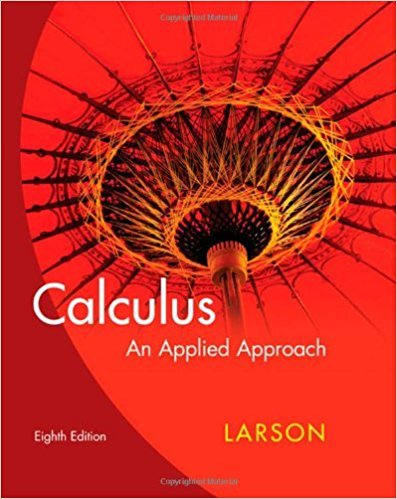×
×

# Solutions for Chapter 3.8: Differentials and Marginal Analysis## Full solutions for Calculus: An Applied Approach | 8th Edition

ISBN: 9780618958252Solutions for Chapter 3.8: Differentials and Marginal Analysis

Solutions for Chapter 3.8
4 5 0 421 Reviews
20
0
##### ISBN: 9780618958252

Since 45 problems in chapter 3.8: Differentials and Marginal Analysis have been answered, more than 21908 students have viewed full step-by-step solutions from this chapter. Calculus: An Applied Approach was written by and is associated to the ISBN: 9780618958252. Chapter 3.8: Differentials and Marginal Analysis includes 45 full step-by-step solutions. This textbook survival guide was created for the textbook: Calculus: An Applied Approach , edition: 8. This expansive textbook survival guide covers the following chapters and their solutions.

Key Calculus Terms and definitions covered in this textbook
• Acute triangle

A triangle in which all angles measure less than 90°

• Derivative of ƒ

The function defined by ƒ'(x) = limh:0ƒ(x + h) - ƒ(x)h for all of x where the limit exists

• Differentiable at x = a

ƒ'(a) exists

• Focal length of a parabola

The directed distance from the vertex to the focus.

• Horizontal translation

A shift of a graph to the left or right.

• Implied domain

The domain of a function’s algebraic expression.

• Infinite limit

A special case of a limit that does not exist.

• Interquartile range

The difference between the third quartile and the first quartile.

• Inverse sine function

The function y = sin-1 x

• Mathematical model

A mathematical structure that approximates phenomena for the purpose of studying or predicting their behavior

• NDER ƒ(a)

See Numerical derivative of ƒ at x = a.

• Paraboloid of revolution

A surface generated by rotating a parabola about its line of symmetry.

• Polar distance formula

The distance between the points with polar coordinates (r1, ?1 ) and (r2, ?2 ) = 2r 12 + r 22 - 2r1r2 cos 1?1 - ?22

• Principle of mathematical induction

A principle related to mathematical induction.

Any one of the four parts into which a plane is divided by the perpendicular coordinate axes.

• Solve an equation or inequality

To find all solutions of the equation or inequality

• Standard unit vectors

In the plane i = <1, 0> and j = <0,1>; in space i = <1,0,0>, j = <0,1,0> k = <0,0,1>

• Tree diagram

A visualization of the Multiplication Principle of Probability.

• Vertical stretch or shrink

See Stretch, Shrink.

• z-axis

Usually the third dimension in Cartesian space.

×